# slicesample

## Syntax

``rnd = slicesample(initial,nsamples,"pdf",pdf)``
``rnd = slicesample(initial,nsamples,"logpdf",logpdf)``
``rnd = slicesample(___,Name,Value)``
``[rnd,neval] = slicesample(___)``

## Description

example

````rnd = slicesample(initial,nsamples,"pdf",pdf)` returns a Markov chain of `nsamples` random samples from the density function `pdf` using the slice sampling method (see Algorithms). `initial` is a scalar or vector specifying the initial point from which the function starts sampling. ```

example

````rnd = slicesample(initial,nsamples,"logpdf",logpdf)` generates `nsamples` random samples from the log density function `logpdf`. During sampling, `slicesample` uses `logpdf` to evaluate the logarithm of the density function directly, instead of first evaluating the density function and then taking the log. You can use this syntax if the density function is already in logarithmic form (see Tips). ```

example

````rnd = slicesample(___,Name,Value)` specifies options using one or more name-value arguments in addition to any of the input argument combinations in previous syntaxes. For example, you can specify the burn-in period, the sample discard interval, and the starting interval width.```

example

````[rnd,neval] = slicesample(___)` additionally returns the average number of function evaluations per sample that occurred in the slice sampling.```

## Examples

collapse all

Generate random samples from a univariate density function using `slicesample`.

Create a function handle to the normal probability density function.

```rng default % For reproducibility mean = 4; sigma = 2; % Standard deviation pdf = @(x) normpdf(x,mean,sigma); ```

Generate 1000 samples from `pdf` with no burn-in period. Use an initial value of 4.

```initial = 4; nsamples = 1000; sampleValues = slicesample(initial,nsamples,"pdf",pdf);```

Plot the sample value chain.

```plot(sampleValues,":*") grid on xlabel("Sample Number");```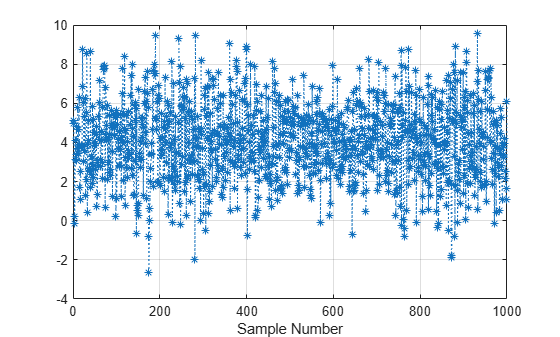Plot a normalized histogram of the sample values. Superimpose the normal probability density function on the histogram.

```h = histogram(sampleValues,25,"Normalization","pdf"); hold on xgrid = linspace(h.BinLimits(1),h.BinLimits(2),200); plot(xgrid,normpdf(xgrid,mean,sigma),"r","LineWidth",2) hold off```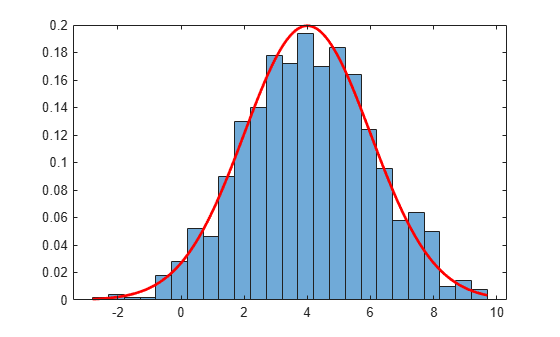The histogram shows that the resulting random samples from `normpdf` have an approximately normal distribution with a mean of 4 and standard deviation equal to 2.

Generate random samples from a density function in logarithmic form using `slicesample`.

Create a function handle to the logistic probability density distribution function with a mean of 0 and standard deviation equal to 1.

`pdf = @(x) exp(x)./(1+exp(x)).^2;`

Create a function handle to the loglogistic distribution function.

`logpdf = @(x) x-2*log(1+exp(x));`

Generate 1000 samples from `logpdf` with no burn-in period. Use an initial value of 0.

```rng default % For reproducibility initial = 0; nsamples = 1000; sampleValues = slicesample(initial,nsamples,"logpdf",logpdf);```

Plot the sample value chain.

```plot(sampleValues,":*") grid on xlabel("Sample Number");```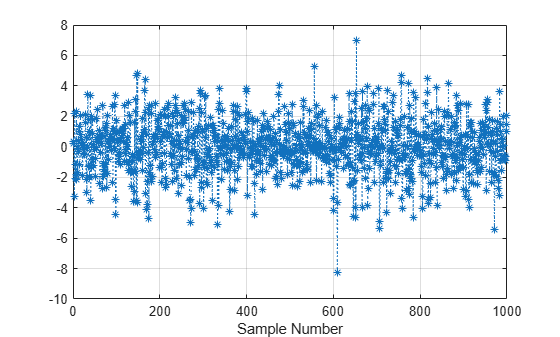Plot a normalized histogram of the sample values. Superimpose the logistic distribution function on the histogram.

```h = histogram(sampleValues,"Normalization","pdf"); hold on xgrid = linspace(h.BinLimits(1),h.BinLimits(2),200); plot(xgrid,pdf(xgrid),"r","LineWidth",2) hold off```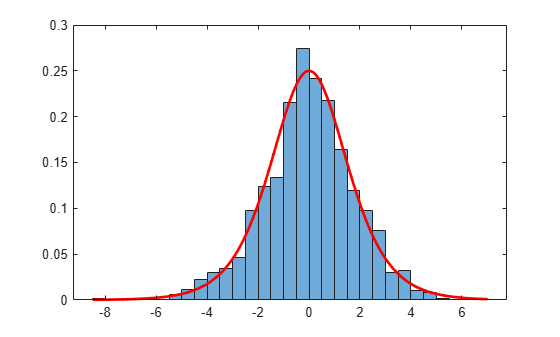The histogram shows that the random samples generated from `logpdf` have an approximately logistic distribution.

Generate random samples from a multimodal density function using `slicesample`.

Define a function proportional to a multimodal density. The function does not need to integrate to 1.

```rng default % For reproducibility pdf = @(x) exp(-x.^2/2).*(1 + (sin(3*x)).^2).* ... (1 + (cos(5*x).^2));```

Generate random samples from `pdf`, starting with an initial value of 1. Discard the first 1000 samples, and keep every fifth sample thereafter. The output sequence has 2000 samples.

```N = 2000; sampleValues = slicesample(1,N,"pdf",pdf,"burnin",1000,"thin",5);```

Plot a normalized histogram of the sample values. Superimpose the density function on the histogram.

```h = histogram(sampleValues,50,"Normalization","pdf"); hold on xgrid = linspace(h.BinLimits(1),h.BinLimits(2),1000); area = integral(pdf,-5,5); y = pdf(xgrid)/area; plot(xgrid,y,"r","LineWidth",2) hold off```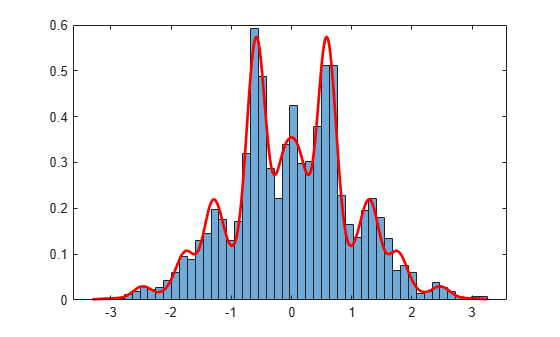The samples provide a good fit to the multimodal density function, indicating that the specified `burnin` value is adequate.

Examine the stationarity of a random sample sequence generated by `slicesample`, and return the average number of function evaluations per sample.

Define a function proportional to a multimodal density.

```rng default % For reproducibility pdf = @(x) exp(-x.^2/2).*(1 + (sin(3*x)).^2).* ... (1 + (cos(5*x).^2));```

Plot the density function.

```x = linspace(-4,4,500); plot(x,pdf(x),"r","LineWidth",2) xline(0);```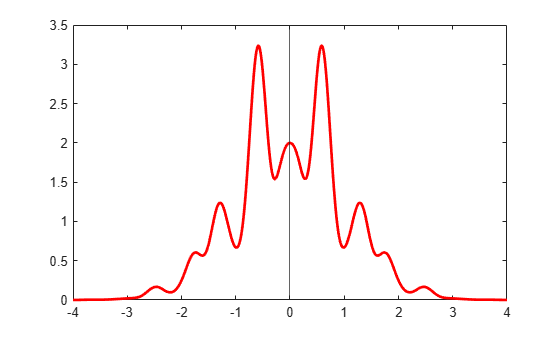The density function is symmetric about the origin and, therefore, has an expectation value of zero.

Generate 100 samples from the density function with no burn-in period. Use an initial value of 20 and a sample width of 15.

```nsamples = 100; initial = 20; width = 15; burnin = 0; [sampleValues,neval] = slicesample(initial,nsamples, ... "pdf",pdf,"width",width,"burnin",burnin); neval```
```neval = 4 ```

The `slicesample` function makes an average of `neval` function evaluations per generated sample.

Plot the sample sequence values and the moving average value. Use a moving average window width of 10 samples.

```plot(sampleValues,":*") yline(0); xlabel("Sample Number"); movavg = movmean(sampleValues,10); hold on plot(movavg,"LineWidth",2) hold off```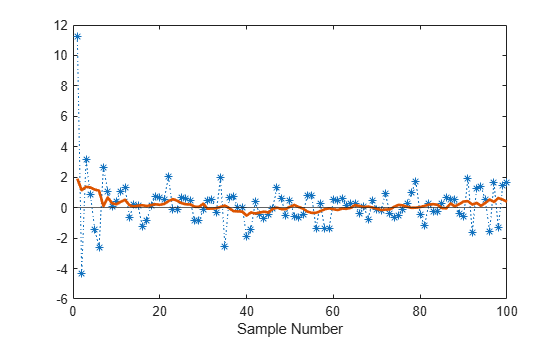The mean value of the sequence approaches stationarity and the expected (zero) value of the density function after approximately seven samples. You can attain stationarity faster by specifying different values for `initial` and `burnin`.

## Input Arguments

collapse all

Initial point to start sampling from, specified as a scalar or vector. The initial point must lie within the domain of the density function. `pdf(initial)` must be a strictly positive scalar. Each output sample has `numel(initial)` dimensions.

Example: 1

Data Types: `single` | `double`

Number of output samples generated by `slicesample`, specified as a positive integer.

Example: 1000

Data Types: `single` | `double`

Density function to generate samples from, specified as a function handle. The `pdf` function must accept an argument of the same type and size as `initial`. The `pdf` function can be unnormalized, meaning it does not need to integrate to 1.

Example: `@normpdf`

Data Types: `function_handle`

Logarithmic density function to generate samples from, specified as a function handle. `logpdf` must accept an argument of the same type and size as `initial`. The `logpdf` function can be the logarithm of an unnormalized density function. During sampling, `slicesample` uses `logpdf` to evaluate the logarithm of the density function directly, instead of first evaluating the density function and then taking the log.

Use this argument if the density function is already in logarithmic form. Doing so can improve numerical stability if `logpdf` has a more simple, closed form than `log(pdf)`(see Tips).

Example: `@(x) log(exppdf(x))`

Data Types: `function_handle`

### Name-Value Arguments

Specify optional pairs of arguments as `Name1=Value1,...,NameN=ValueN`, where `Name` is the argument name and `Value` is the corresponding value. Name-value arguments must appear after other arguments, but the order of the pairs does not matter.

Example: `slicesample(1,4000,"pdf",pdf,burnin=1000,thin=5)` generates 4000 output samples from the density function `pdf`, with an initial point equal to 1. The first 1000 samples are discarded, and every fifth sample is kept thereafter.

Before R2021a, use commas to separate each name and value, and enclose `Name` in quotes.

Example: `slicesample(1,4000,"pdf",pdf,"burnin",1000,"thin",5)` generates 4000 output samples from the density function `pdf`, with an initial point equal to 1. The first 1000 samples are discarded, and every fifth sample is kept thereafter.

Number of samples to generate and discard before generating the samples to return, specified as a nonnegative integer. `slicesample` generates a Markov chain of samples whose stationary distribution is proportional to the density function `pdf`. Set `burnin` to a high enough value for the Markov chain to approximately reach a stationary state after generating `burnin` samples. For an example, see Analyze Slice Sampler Output and Performance.

Data Types: `single` | `double`

Sample discard interval, specified as a positive integer. `slicesample` discards every `thin – 1` samples and keeps the next one. The slice sampling algorithm is a Markov chain, so the samples are serially correlated. To reduce the amount of serial correlation, specify a larger value for `thin`.

Data Types: `single` | `double`

Starting interval width around the current sample, specified as a nonnegative scalar or a vector of positive values. `slicesample` iteratively expands the interval around the current random value by `width` until both ends are outside the slice.

• If `width` is a scalar and the samples have multiple dimensions, `slicesample` uses `width` for each dimension.

• If `width` is a vector, it must have the same length as `initial`.

Data Types: `single` | `double`

## Output Arguments

collapse all

Random sample chain, returned as an `nsamples`-by-`numel(initial)` matrix. Each row contains one sample.

Average number of function evaluations per sample, returned as a scalar. `neval` includes the `burnin` and `thin` evaluations (if specified), in addition to the evaluations of samples returned in `rnd`. Therefore, if you specify `burnin` and `thin` values, the total number of function evaluations is `neval*(nsamples*thin + burnin)`. Otherwise, the total number of function evaluations is `neval*nsamples`.

## Tips

• Use `logpdf` instead of `pdf` for density functions where numerical overflow or underflow errors might occur.

• There are no definitive guidelines for determining appropriate values for `thin` and `burnin`. Specify starting values for `thin` and `burnin` and then increase them, if necessary, to achieve the requisite sample independence and similarity to the target density function.

• If the step-out procedure (see Algorithms) fails, by exceeding the maximum number of 200 function evaluations while generating a sample, you might have to experiment with different values of `width`. If `width` is too small, the algorithm might implement an excessive number of function evaluations to determine the extent of the slice. If `width` is too large, the algorithm tries to decrease the width to an appropriate size, which might also result in a large number of function evaluations. The `neval` output argument returns the average number of function evaluations per sample.

## Algorithms

Slice sampling is a Markov Chain Monte Carlo (MCMC) algorithm that samples from a distribution with an arbitrary density function, known only up to a constant of proportionality. This situation can arise when sampling is needed from a complicated Bayesian posterior distribution whose normalization constant is unknown. The algorithm does not generate independent samples, but rather a Markov chain whose stationary distribution is the target distribution.

The `slicesample` function draws samples from the region under the density function using a sequence of vertical and horizontal steps. First, the algorithm selects a height at random between 0 and the value of the density function f(x) at a specified initial value of x. Then, the algorithm selects a new x value at random by sampling from the horizontal “slice” that contains all x values where the density function is greater than the selected height. The `slicesample` function uses a similar slice sampling algorithm in the case of a multivariate distribution.

Choose a density function f(x) that is proportional to the target density function, and do the following to generate a Markov chain of random numbers:

1. Select an initial value x(t) that lies within the domain of f(x).

2. Draw a real value y uniformly from (0, f(x(t))), thereby defining a horizontal “slice” as S = {x: y < f(x)}.

3. Find an interval I = (L, R) around x(t) that contains all or much of the “slice” S.

4. Draw a new point x(t + 1) from within this interval.

5. Increment t by 1, and repeat steps 2 through 4 until you get the number of samples you want.

`slicesample` uses the slice sampling algorithm of Neal. For numerical stability, `slicesample` operates on the logarithm of the `pdf` function (unless the `logpdf` argument is specified). `slicesample` uses Neal's “stepping-out” and “stepping-in” method to find the interval I containing the slice S. The algorithm tries a maximum of 200 step-out and 200 step-in iterations when generating each sample.

Nearby points in the chain tend to have values that are closer together than they would be from a sample of independent values. For many purposes, the entire set of points can be used as a sample from the target distribution. However, when this type of serial correlation is a problem, the `burnin` and `thin` name-value arguments can help reduce the correlation.

## Alternative Functionality

 Neal, Radford M. "Slice Sampling." The Annals of Statistics Vol. 31, No. 3, pp. 705–767, 2003. Available at https://doi.org/10.1214/aos/1056562461.